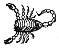# What is the point of intersection?

Elizabeth A Williams falls off a logSeveral months ago, one of my A-level students shared a puzzle with me. It’s a neat puzzle in its own right, but what came out of our conversation is even neater. My student made a fantastic insight into understanding what a logarithm was trying to say.

Let $y=b^x$ where $b > 0$ and $b\neq 1$. Consider the line which passes through the origin and is tangent to this graph at point $T$. What are the coordinates of $T$?This is great fun to slap into your graphing program of choice (all hail Desmos!). Look at $y = 2^x$, $y = 3^x$, maybe $y =(1/2)^x$. Draw the line $y = mx$ and twiddle its gradient until the line just meets the exponential. The program will give a good approximation to the coordinates of $T$, and voilà, you’re off and away to happy sandboxing and conjecturing.

Pause the article here to have a play for yourself.It’s a pretty neat result. But—cracks knuckles—there is much satisfaction to be had in proving a conjecture. My student and I brandished our pencils, and this is what we wrote.

Take $y = 3^x$. The tangent line through the origin intersects the graph at point $T$ with coordinates $(t,\ 3^t)$. There are two ways to calculate the gradient of this tangent line.

Because the line goes through $(0,\ 0)$ and $(t,\ 3^t)$, $\Delta y/\Delta x = (3^t – 0)/(t – 0) = 3^t/t$.

Also, as $y = 3^x$, $\mathrm{d}y/\mathrm{d}x = \ln 3 \times 3^x$. At point $T$, the gradient of the tangent is $\ln 3 \times 3^t$.

Equating these two expressions and solving for $t$ gives $t = 1/\ln 3$. Thus the coordinates of $T$ are $\left(1/\ln 3 , 3^{1/\ln 3}\right)$.

In general, for $y=b^x$, the coordinates of $T$ are $\left(1/\ln b , b^{1/\ln b}\right)$.

Ta-dah! We sat back in our chairs.

Except… what kind of number is $3^{1/\ln 3}$?! Our conjecture had strongly hinted at what to expect, and this wasn’t quite it.

I leaned forward again, scratching my pencil across the paper to find a simplification. My student remained still, regarding the expression $3^{1/\ln 3}$ thoughtfully. Then he observed, “That’s $\mathrm{e}$. It’s just $\mathrm{e}$.”

! How did he recognise it on sight?

One of my mathematical mantras is logs are powers (ommm). That is, $\log_bc$ is the power to which you raise $b$ in order to get $c$. The number $\log_bc$ is named by the description of what it does, much like how writing $\sqrt{5}$ means the number such that when you square it, you get 5. We’re identifying a specific number by its property rather than by its explicit value. This mantra directly generates the delightful construction
$$b^{\log_bc}$$
which now clearly shakes out as $c$. See? $\log_bc$ is the power you raise $b$ to, in order to get $c$. We’ve raised $b$ to that very power, so what do we get? $c$. Hurrah!

My student had taken this mantra to heart. And then he took it one step further. He reasoned as follows.

We know, by the mantra, that $\mathrm{e}^{\ln3} = 3$.

Also, the expression $3^{1/\ln 3}$ means take the $(\ln 3)^{\text{th}}$ root of $3$.

If we take the $(\ln 3)^{\text{th}}$ root of both sides of $\mathrm{e}^{\ln3} = 3$, then on the LHS, we are left with just $\mathrm{e}$.

Hence, $3^{1/\ln 3}$ equals $\mathrm{e}$.

I think this is a beautiful insight. It comes from viewing a power as a root and then seeing the power of that root, as it were. Until my student pointed it out, I hadn’t realised this elegance.